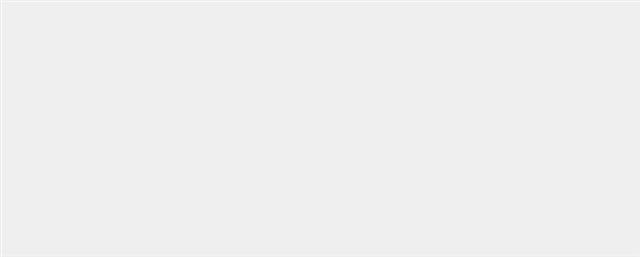#### 設計說明：

(1) 請撰寫三個方法計算圓形、三角形和長方形圖形的面積，並輸出總面積。

(2) 假設圓的半徑 = 5，PI = 3.1415926，請寫出 calCircle 函數計算圓面積。

(3) 圓面積計算公式：半徑平方 * 圓周率 (PI)。

(4) 三角形的底 = 10，高 = 5，請寫出 calTriangle 函數計算三角形面積。

(5) 三角形面積計算公式：底 * 高 / 2。

(6) 假設長方形的長 = 5，寬 = 10，請寫出 calRectangle 函數計算方形面積。

(7) 長方形面積計算公式：長 * 寬。

(8) 圖形面積 = 圓面積 + 三角形面積 + 長方形面積。

(9) 顯示如執行結果參考畫面。

JPD01.java 檔案下載

#### 程式碼：

``````import java.util.Scanner;
public class JPA01 {
public static void main(String args[]) {
double totalarea;
System.out.printf("圓形面積為：%f \n",calCircle(5));
System.out.printf("三角形面積為：%f \n",calTriangle(10, 5));
System.out.printf("方形面積為：%f \n",calRectangle(5, 10));
totalarea = calCircle(5) + calTriangle(10, 5) + calRectangle(5, 10);
System.out.printf("此圖形面積為：%f \n",totalarea);
}
public static double calCircle(int r) {
double PI = 3.1415926;
return PI * Math.pow(r, 2);
}
public static double calTriangle(int b, int h) {
return b * h / 2;
}
public static double calRectangle(int l, int w) {
return l * w;
}
}``````

JPA01.java 檔案下載

### TQC+ 物件導向程式語言 Java 6 第一類：基本認識